# Test: Table Charts (Easy)

## 5 Questions MCQ Test Logical Reasoning (LR) and Data Interpretation (DI) | Test: Table Charts (Easy)

Description
This mock test of Test: Table Charts (Easy) for Banking Exams helps you for every Banking Exams entrance exam. This contains 5 Multiple Choice Questions for Banking Exams Test: Table Charts (Easy) (mcq) to study with solutions a complete question bank. The solved questions answers in this Test: Table Charts (Easy) quiz give you a good mix of easy questions and tough questions. Banking Exams students definitely take this Test: Table Charts (Easy) exercise for a better result in the exam. You can find other Test: Table Charts (Easy) extra questions, long questions & short questions for Banking Exams on EduRev as well by searching above.
QUESTION: 1

### Q. Study the following table and answer the questions. Number of Candidates Appeared and Qualified in a Competitive Examination from Different States Over the Years.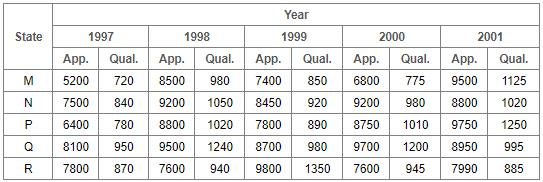Total number of candidates qualified from all the states together in 1997 is approximately what percentage of the total number of candidates qualified from all the states together in 1998?

Solution:

Required percentage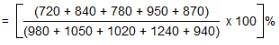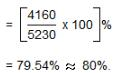QUESTION: 2

### Study the following table and answer the questions. Number of Candidates Appeared and Qualified in a Competitive Examination from Different States Over the Years.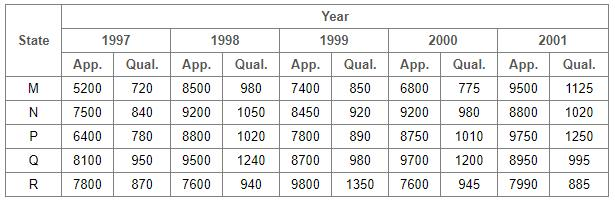What is the average candidates who appeared from State Q during the given years?

Solution:

Required average =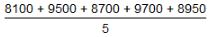= 44950/5
= 8990

QUESTION: 3

### Study the following table and asnwer the questions. Number of Candidates Appeared and Qualified in a Competitive Examination from Different States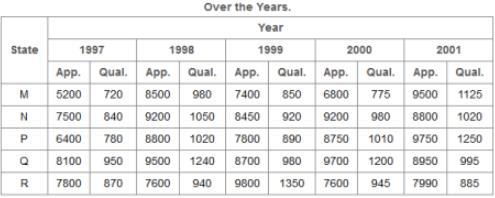In which of the given years the number of candidates appeared from State P has maximum percentage of qualified candidates?

Solution:

The percentages of candidates qualified to candidates appeared from  State P during different years are: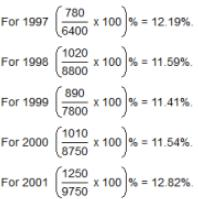∴ Maximum percentages is for the year 2001.

QUESTION: 4

Study the following table and answer the questions.
Number of Candidates Appeared and Qualified in a Competitive Examination from Different States.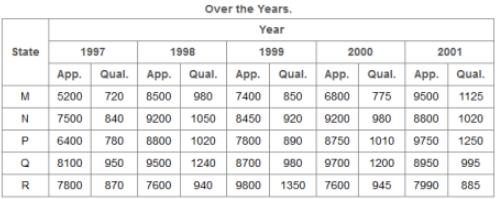What is the percentage of candidates qualified from State N for all the years together, over the candidates appeared from State N during all the years together?

Solution:

Required percentages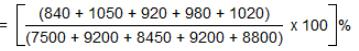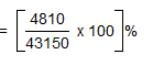= 11.15%

QUESTION: 5

Study the following table and answer the questions.
Number of Candidates Appeared and Qualified in a Competitive Examination from Different States Over the Years.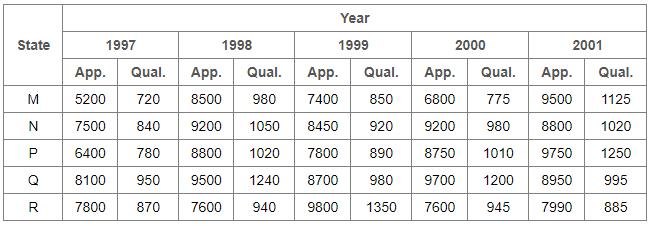The percentage of total number of qualified candidates to the total number of appeared candidates among all the five states in 1999 is?

Solution:

Required percentage =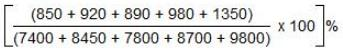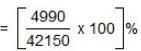= 11.84%